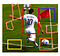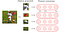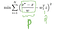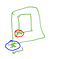# Object Detection — RCNN

This chapter covers the content discussed in the Object Detection module of the Deep Learning course offered on the website: https://padhai.onefourthlabs.in

Overview:

An example of Object Detection:

In Image Classification, we are given an image and the model predicts the class label for example for the above image as the input, model would predict that this is a car i.e the input is an image and the output is a class label whereas in the case of Object Detection the input is an image and the output is the label Car as well as the exact bounding box containing car.

So, the model gives us the coordinates of the bounding rectangle. It could return back the coordinates of the lower left-most point of the rectangle and its width and height.

In a similar way, we could also do multiple object detection in the same image.

# A typical pipeline for Object Detection:

Let’s say this is the image given to the model:

And our goal is to identify all objects in it, we can see that there are 3 objects, there is this person, then there is a flag and then there is a ball and the other yellow bounding box there is no object over there, there is nothing in that region so the model should be able to identify/say that as well.

Region Proposal Phase

Regional Proposal Phase is one stage in the pipeline where some regions in the image are proposed as the regions which might contain the object(s), (this is a big image given to model). Here in the above image, we have shown only 4 regions but in actual practice, there would be many more regions of different shapes, sizes and aspect ratio:Multiple regions of different shape, size, aspect ratio in the input image

These are the regions where there might be an object, so it tries to predict whether the proposed regions actually contains an object and if so what is the class/label of the object.

Once the regions are proposed, then we will crop out(we give the co-ordinates of the lower-left corner and the width and the height of rectangle based on which it will crop this region from the input image and make a new image; so all of the regions/images would be cropped to the same size(let’s say this size is p, q)) each of these regions:

Now from these cropped images, we need to do some sort of feature extractions:All the vectors would be of the same dimensions as we ensure that all the regions when cropped out have the same dimensions.

Let’s say we crop all the images to be of the size 30 X 30 so ‘d’ is 30(30-dimensional vector) in this case and each of the cropped images would then be a 900-dimensional vector(30 X 30 vector/matrix when flattened out) and we want to do the classification on that.

Our input ‘x’ in this case would be a vector containing 900 values and the output ‘y’ could be any of the class out of the total no. of classes let’s say total 4 classes are there so y could be Class A, Class B, …, Class D.

We could do this classification using one layer neural network or a deep neural network or using a CNN as well.

We can think of the Classifier as the last layer of the neural network where we have passed the input through multiple layers of a neural network, each layer gives a different feature representation. If we are using a CNN, then at each layer some convolutional operation would be there and we get feature map as the output which we can think of as the feature representation and the last layer’s output then we feed it to a classifier, we can think of it as a single output layer which would be softmax of course as we want the probability distribution over the 4 classes(in this case) and in each of cases we know what the true class is, so we can think of training this using the Cross-Entropy Loss.

So, there are 3 stages:

i.) Region Proposal Stage

ii.) Feature Extraction Stage

iii.) Classifier Stage

The regions proposals that we have are not perfect; if we look at the below image, we had proposed this red region(for person class label) but that’s not the complete region in which the person is

The true region(for person class label) should be something like in the below image(purple bounding box) which covers the entire person from head to toe(this region we can extend wider as well to cover the arms):

So, then what happens is whatever red box we have given you which had some values a, b, w, h(proposed box coordinates) these are not the true values because there is scope for improvement, we have not localized the person in the image completely, so instead of these values we want to predict next set of values a’, b’, w’, h’(a-dash, b-dash, w-dash, h-dash)

So, a, b, w, h are the values what we have proposed and based on this proposal we want to do a new prediction about what is the true values because we know that in our training set when we will be given this image, this entire person from head to toe will be marked as a single box whereas whatever we had proposed is an incorrect box.

So, whenever we propose a region, we need to do two things in that:

1. Tell you what is the object inside that box
2. And also tell you whether the scope was stretching this region so that it becomes better.

So, let’s look at this problem which is a regression problem:

Think of the regions as the cropped image(we know the 4 dimensions i.e a, b, w, h for each image); using this image we want to predict 4 new values

Proposed values in the above image represents the Input values to the model; the model would predict some value which are represented by Predict in above image and then we have the True values(true box which a human can mark in the image) and this true values/output we can represent as y and the predicted values by y_hat and the image defined by the proposed values represent our input x.

Given y_hat and y, we can define a loss function and we can train the model so that the model’s output is close to the true output.

Input x is an image which is defined by 4 coordinates(these co-ordinates are not the input) and the output is going to be 4 values a’, b’, w’, h’ and the true output is a*, b*, w*, h* which is also a 4-dimensional vector.

So, the loss function, in this case, would be the squared error loss

So, everything else remains the same the only thing that we will change is the Loss function(last layer); instead of Cross-Entropy Loss, we use the Squared Error Loss. Once we compute the derivative of the Loss function with respect to last layer everything else remains the same, it would be the same backpropagation algorithm that we have seen in the case of the classification problem.

We update the respective weights/biases as per the usual formula/method:

In the case of regression cases, we don’t need any activation function. The activation hL would be the same as the pre-activation aL. We don’t need the activation function as the output would be a real value and no need to normalize the output as opposed to the classification where we pass through the output of final layer through the softmax activation to compute the probability distribution(true output is also a probability distribution where the entire mass is on one class label).

More Clarity on Regression

There are two main differences in the Regression problem as compared with the Classification problem:

1. The loss function that we use is the Squared Error Loss in case of Regression problems whereas we use the Cross-Entropy Loss in case of the Classification problem. For CE Loss, we just need y and y_hat whereas for Squared Error Loss we will be requiring all the ‘k’ predicted values as well as the ‘k’ true values for a ‘k’ dimensional output.
2. The second difference is of the output layer.

Let’s say we are given this ‘d’ dimensional input data related to movie, using this input we can do two tasks here, we can do the classification as whether the user would like this movie or not; we can also do the regression task here where we would be predicting the box office collection of the movie.

In both cases, the input is going to be the same; the neural network is going to be the same until the last layer.

Last layer is going to be softmax in case of classification case because the output from the final layer is going to be a set of real values and we want the probability distribution so that these values lie in between 0 and 1 whereas in the case of regression we want a real value as the output and the output from the final layer is also a real value so we could use this output as the final output. Based on this we compute the Loss value and adjust the weights accordingly.

RCNN — Region Proposal

RCNN uses Selective Search for Region Proposals

Given an image, we can do clustering(clustering is a simple technique in which the similar objects are put into the same cluster). So, if we look at the above image, there is this green patch(grass), that entire patch could be one cluster:

Then it has this entire white region here(where the sheep is), that could be one cluster:

The person is wearing some shirt or jacket, based on that it could be one cluster:

This would be a simple technique because these pixels would have the same color and texture(we can use OpenCV for doing this) and there would be some threshold, so if the similarity between two regions is above some threshold then we make them into one cluster. That’s exactly what this Selective Search does.

In the above image(1st row, last pic) we have only 5 clusters so that means in this case we are being more liberal/lenient in combining things, even if there similarity is small we are still combining them.

We would have many different thresholds for different levels.

At the lowest level, each pixel would be in its own cluster, we are saying it's not similar to anything else.

At the next level, two pixels would be merged if their similarity level is above some pre-defined threshold.

So, we get these different regions as

So, multiple boxes/regions are passed to the model and the classification engine needs to decide whether there is an object or not and the regression engine needs to ensure whether the bounding box needs to be stretched to cover the complete object.

RCNN — Feature Extraction

Once we have proposed the region

we need to crop it out to form the mini images

then we scale this cropped image so that all the cropped images are of the same size

So, we pass this scaled cropped image to the CNN where multiple filters of different dimension would be convoluted over this input image to extract features from it and all these layers would be stacked up and passed through the fully connected layers and whatever be the output at the fully connected layer that we extract as the feature representation for this image(we could take the representation from any of the layers but the conventions is to take it from the last second or last fully connected layer).

We can use any of the CNNs for feature extraction. The first thing we need to do is to train the CNN on the ImageNet dataset using the Standard Cross-Entropy loss, so the result of this would be that the filters will arrive at certain weight configuration and at the same time these intermediate layers will learn some meaningful representation of the image. If they are learning the important parts of an image only then we can say that the final classification layer would do a good job. So, being trained on the ImageNet dataset, it is able to learn a good representation of a wide variety of classes and the same classes we expect to be in object detection also(as ImageNet has 1000 classes).

So, we take the final representation from the fully connected layer.

So, that’s what feature extraction does, for a given image, instead of using raw pixels we use the feature representation given by the fully connected layer of the pre-trained CNN. Based on this representation we will do two tasks — one is classification and the other is the regression.

RCNN — Classification

The way RCNN does the classification is that uses a SVM. Our training data consists of the bounded box around the objects in the image and their corresponding label for example:

And instead of the image as the input to the classifier, we have its feature representation as the input to the classifier.

In the original paper, they used SVMs instead of Neural Network but we could use any of the network/classifiers. They used as many SVMs as the number of classes.

So, we pass the feature representation through all the SVMs and each SVM would give the confidence value of the object in the image belonging to that class.

We could also model it using a neural network:

We pass the final feature representation through one, two or more layers and then through the final softmax layer which would give the probability distribution and the way we train this network is that for each image in the input we would 2–3 object instances/entities in it and the ground truth as well so based on this data from all the images we will be able to train this neural network.

RCNN — Regression

So, this is the last stage in our task, which is just stretching the bounding box. We have proposed some regions during Selective Search but those regions may not be perfect

We represent the box using its coordinates of the lower-left corner(x, y) and its width(w) and height(h).

The input is the proposed box(coordinates) and the model output would be the predicted coordinates of the box and there would be true coordinates as well:

Proposed coordinates: x, y, w, h

Predicted Coordinates: x_hat, y_hat, w_hat, h_hat

True Coordinates: x*, y*, w*, h*

We want the model to predict the coordinates as close as to the True coordinates. And in general, instead of predicting the four values, we can actually predict the difference between the values.

Difference between the True and the proposed coordinates:

(x* — x), (y*- y), (w* — w), (h* — h)

And we want the model to predict this difference(above four values) that’s what we make our regression task. If we know this difference, we get to know how much to move x to get to x*. So, instead of predicting x*, it makes more sense to predict the difference because that would be a smaller quantity and we just want to find how much to displace the proposed box instead of finding the coordinates from scratch.

We want to predict the normalized difference( (x* — x)/w ).

Let ( (x* — x)/w ) = p

and ( (y* — y)/h) = q

So, given x, y if we can predict p and q then we can find the value of x* and y*.

So, p and q are the true quantity that we want to predict.

We are given a cropped image/box as the input, which we pass through the CNN and we get a vectorial representation for the image(let’s say z). z is a ‘d’ dimensional vector and we need a real output, so we use a very basic feed-forward network with just one layer having weights represented by w1(w1 is also a ‘d’ dimensional vector). So, we train the model in such a way that the weights are adjusted so that the Predicted value is as close to the true value.P is the true quantity and P_hat is the quantity predicted by the modelWe have the value for the proposed coordinates(in red) and we have the true coordinated(in blue), we can compute the difference between the two

P_hat would be a function of z(and the function is just ( w1(transpose)z) ) where z is a representation of the input image.

Our goal is to train the model such that it minimizes the difference between P and P_hat for all the training examples.

The same thing we repeat for the y coordinate, w and h also.

RCNN — Training

Region Proposal — There is no training required for Region Proposal, it is just a simple clustering algorithm(we look at the pixels and club them together based if they have a similarity greater than a certain threshold and we use features like color, texture, etc. to find out the similarity).

Feature Extraction — The training at this stage happens using the ImageNet data, we use the pre-trained model(there is no training required here).

Our training would be like an image with a bounding box and the label corresponding to the object/entity enclosed in the bounding box.

Suppose our VGG-Net(used for Image Net) is used on images of dimensions (3 X 300 X 300) and in our training data we have the marks/bounding box, we could take the bounding box and convert that to a 300 X 300 image along with 3 channels so it would be of dimensions (3 X 300 X 300) and we could do this for all the boxes that are marked in the training data.

Now we can load the model of VGG-Net which was trained on ImageNet(for some no. of epochs), we get the configuration of the weights of this model, now we feed it with the data(object detection dataset and not from the ImageNet dataset) that we have here(bounding boxes converted to images), so we start with the configuration of the weights as per ImageNet and try to refine them further so that for this training data the total Cross-Entropy Loss is minimized, we backpropagate this loss and fine-tune this VGG-Net model.

Classifier Stage: The goal of the classifier is that given a cropped and stretched(as we stretch it to 3 X 300 X 300) image, we pass it through a neural network(say 2 layers fully connected network) and we have say some k (20 in the below image) classes at the output

W1, W2, W3 are the weights that we have to learn. So, we have the training data(cropped and stretched image and their corresponding labels) which we can use to train our model(part in blue in the below image).

Regression Stage: We run the Selective Search on all the training data that we have and we get the proposed regions/boxes(coordinates) and for the same data we have the True boxes/coordinates, using these coordinates we try to find the normalized difference between the true coordinates and the proposed coordinates.

Because selective search does the clustering at different levels, so it might be the case that both boxes(overlapping blue and black boxes in the below image) might come up in the proposal by the selective search

So, the question is what we take as the proposed box here and the true box here because both these proposed boxes are overlapping with the same pink box, so the way we deal with this is that we compute the overlap between the proposed box and the true box and if the overlap is more than 50% then we consider this as the proposed box; then we take this proposed box and try to map it with the corresponding true box and train our model to do that.

A drawback of RCNN:

One drawback of RCNN is that the number of computations are very high, for each input image, it would propose some 2000 regions and then for each of the region we crop it and resize it then do the classification and regression task on it. So, it is computationally expensive.

# Summary:

So, given an image, we want to find the bounding boxes around the object in the image as well as the class of the object that lies inside the bounding box. And the other phase that we had was the Region Proposal Phase, so in RCNN region proposal came from the Selective Search which is essentially clustering neighborhood(similar pixels together) based on their texture and color and we make the bounding box(rectangular) around this cluster.

Then we take the cropped portions of the proposed regions, resize it(as required), pass it through a CNN(VGG Net) to extract the useful features, then these feature representation acts as the input to the Classification engine which helps to predict the Label of the object and the other task is of regression to predict the true coordinates of the box(w, h, x, y).